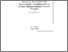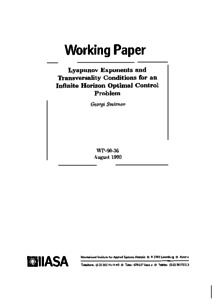# Lyapunov Exponents and Transversality Conditions for an Infinite Horizon Optimal Control Problem

Smirnov, G. (1990). Lyapunov Exponents and Transversality Conditions for an Infinite Horizon Optimal Control Problem. IIASA Working Paper. IIASA, Laxenburg, Austria: WP-90-036Preview
Text
WP-90-036.pdf

## Abstract

The aim of this paper is to derive necessary conditions of optimality for an infinite horizon optimal control problem. The Pontryagin maximum principle for this problem without transversality condition at infinity was derived by Pontryagin. Transversality conditions were derived for some dynamical optimization problems arising from mathematical economics. The presence of an exponential factor in the integral functional is a characteristic of these problems and facilitates consideration to a certain extent. In this case the transversality conditions were formulated in terms of the asymptotic behavior of solutions to an adjoint equation. The problem without an exponential factor was considered by Gani and Wiese under rather restrictive assumptions, and the transversality conditions were given as initial conditions for the solution to the adjoint equation.

It seems quite natural to use stability theory to analyze infinite time optimal control problems. Following this idea we derive the transversality condition at infinity in terms of a Lyapunov exponent of the solution to the adjoint equation. The problem with the exponential factor is considered as well as the problem without it.

Item Type: Monograph (IIASA Working Paper) System and Decision Sciences - Core (SDS) IIASA Import 15 Jan 2016 02:00 27 Aug 2021 17:13 http://pure.iiasa.ac.at/3417View Item